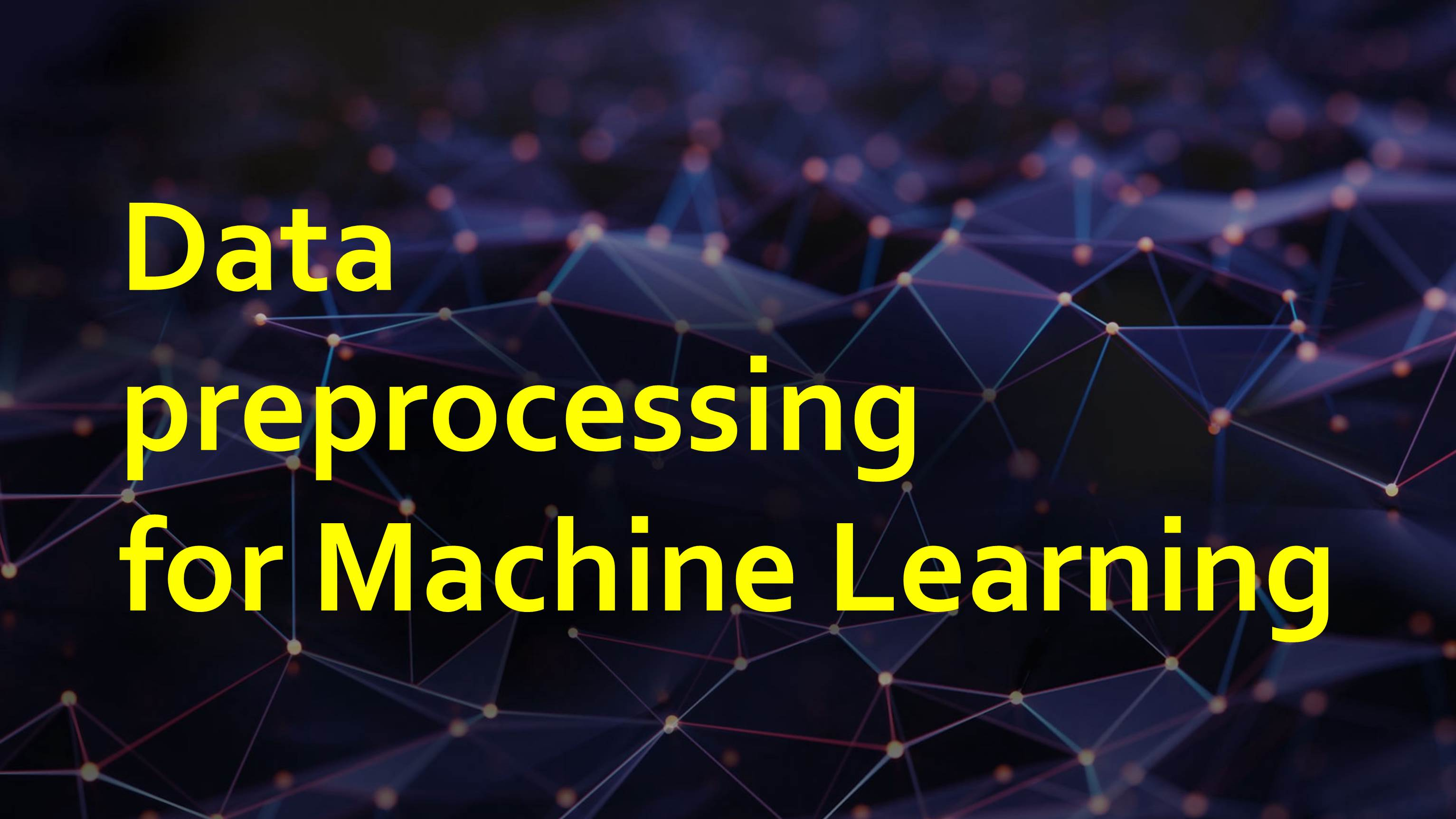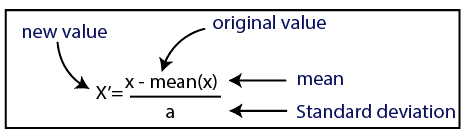/  Technology   /  Data Preprocessing for Machine Learning## Data Preprocessing for Machine Learning

Data preprocessing in Machine Learning refers to the process of preparing the raw data to make it appropriate for a building and training Machine Learning models. In simple words, data preprocessing in Machine Learning nothing but a data mining technique that transforms raw data into an understandable and readable format.

#### Why Data Preprocessing in Machine Learning?

Generally, real-world data is inconsistent, incomplete, inaccurate and often lacks specific attribute values/trends. Here is where data preprocessing comes into scenario. In Machine Learning Data Preprocessing is a crucial step that helps improve the quality of data. Data Preprocessing promotes the extraction of meaningful information from the data.

Data preprocessing involves:

1. Getting the dataset
2. Importing libraries
3. Importing datasets
4. Finding Missing Data
5. Encoding Categorical Data
6. Splitting dataset into training and test set
7. Feature scaling

1. Getting the dataset

A machine learning model completely works on data. So, to build a machine learning model, the first thing we need is a dataset. The dataset is a proper format of collected data for a particular problem.

1. Importing libraries

Python is the most extensively used and also the most preferred library by Data Scientists. So, in order to perform data preprocessing using Python, we need to import some predefined Python libraries. These libraries perform specific job for data preprocessing. The three main Python libraries used for this data preprocessing in Machine Learning are:

• NumPy– NumPy is the essential package for scientific calculation in Python. Therefore, it is used for inserting any kind of mathematical operation in the code. You can also add large multidimensional arrays and matrices in your code.
• Pandas– Pandas is an outstanding open-source Python library for data manipulation and analysis. It is widely used for importing and managing the datasets. It is easy-to-use data structures and data analysis tools for Python.
• Matplotlib– Matplotlib is a Python 2D plotting library that is used to plot any form of charts in Python.

Sample code for importing python libraries.

```import numpy as np
import pandas as pd
import matplotlib.pyplot as mpt```

1. Importing the Datasets

The data which is collected need to be imported at this point of time. Here we use read_csv method to import data which can be found in the pandas library. And also, we need to locate the directory of the csv file (it’s more efficient to keep the dataset in the same directory as your program)

Example code:

`data_set= pd.read_csv('Dataset.csv')`

Extracting dependent and independent variables:

In machine learning, it is important to differentiate the matrix of features (independent variables) and dependent variables from dataset.

Extracting independent variable:

To extract the columns, we will use iloc of pandas (used to fix the indexes for selection) which takes two parameters — [row selection, column selection].

`X = dataset.iloc[:, :-1].values`

In the above line, the first colon(:) is takes all the rows, and the second colon(:) is for all the columns. Here we have used :-1, because we don’t want to consider the last column as it contains the dependent variable. So by doing this, we will extract the matrix of features.

Extracting dependent variable:

To extract dependent variables, again, we use Pandas .iloc[] method.

`y= data_set.iloc[:,3].values`

The above code takes all the rows and only last column. This will give the array of dependent variable.

1. Handling missing data

This will be the next step in data preprocessing. It might create a huge problem for our machine learning model if our dataset contains missing data. Hence it is essential to handle missing values existing in the dataset.

There are two ways to handle missing data. They are

By deleting the particular row: The first way is to normally deal with null values. In this way, we delete the specific row or column which consists of null values. But this is not so efficient and this may lead to loss of information which will not give the accurate output.

By calculating the mean: In this way, we calculate the mean of that column or row which contains any missing value and will put it on the place of missing value. This approach is useful for the features which have numeric data such as age, salary, year, etc.

The library that we will use for the task is called Scikit Learn preprocessing. It contains a class called Imputer which will aid us to take care of the missing data.

`from sklearn.preprocessing import Imputer`

The Imputer class can take few parameters —

1. missing_values: We can give either an integer or “NaN” for it to find the missing values.
2. Strategy: We will calculate the average so we will set it to mean. We can also set it to median or mode as necessary.
3. Axis: We can assign 0 or 1, 0 to impute along columns and 1 to impute along rows.

`imputer = Imputer(missing_values = "NaN", strategy = "mean", axis = 0)`

Now fitting the imputer object to independent variables x.

`imputer = imputer.fit(X[:,1:3])`

Now we replace the missing values with the mean of the column by the method transform.

`X[:, 1:3] = imputer.transform(X[:, 1:3])`

1. Encoding categorical data

Sometimes our data contains text and it may be in the qualitative form. Since machine learning model completely depends on mathematics and numbers, then it gets complicated while building the model, if our dataset has categorical variable. Hence, it is necessary to encode these categorical variables into numbers.

For this purpose, we import the scikit library that we used before. There’s a class in the library called LabelEncoder which is used here.

`from sklearn.preprocessing import LabelEncoder`

The next step is typically to create an object of that class. We will call our object as labelencoder_X.

`labelencoder_X = LabelEncoder()`

A method available in the LabelEncoder class called fit_transform is used.

`X[:,0] = labelencoder_X.fit_transform(X[:,0])`

The above code selects all the rows (:) of the first column (0) and fit the LabelEncoder to it and transform the values. The values will then directly be encoded to 0,1,2,3… accordingly.

In the result text has been replaced with numbers. A problem arises if there are more than two categories.  If one category is assigned with value ‘0’ and another category is assigned ‘2’ value, the machine learning model may assume that there is some correlation between these values which will produce the wrong output. So, to overcome this issue, we will use dummy encoding.

In dummy ecoding we create n number of columns with only 1’s and 0’s to represent whether the category occurs or not.

To achieve the task, we need to import another library called OneHotEncoder.

`from sklearn.preprocessing import LabelEncoder, OneHotEncoder`

As usual we will create an object of that class and assign it to onehotencoder.

```onehotencoder = OneHotEncoder(categorical_features =)
X = onehotencoder.fit_transform(X).toarray()```

1. Splitting the Dataset into the Training set and Test set

Now we need to split our dataset into two sets — a Training set and a Test set. This process will enhance the performance of our machine learning model.

Training Set: A subset of dataset used to train the machine learning model, and we already know the output.

Test set: A subset of dataset used to test the machine learning model, and by using the test set, model predicts the output.

A universal thumb rule is to allocate 80% of the dataset to training set and the remaining 20% to test set.

For this task, we import test_train_split from model_selection library of scikit.

`from sklearn.model_selection import train_test_split`

Now to build our training and test sets, we will create 4 sets— X_train (features for the training data), X_test (features for testing data), Y_train (Dependent variables for training data) , Y_test (Independent variable for testing data).

`X_train, X_test, Y_train, Y_test = train_test_split(X,Y, test_size=0.2)`

In the above code train_test_split takes two parameters arrays (X and Y), test_size (if we give it the value 0.5, meaning 50%, it would split the dataset into half. Since an ideal choice is to allocate 20% of the dataset to test set, it is usually assigned as 0.2)

1. Feature scaling

The final and important step of data preprocessing is to apply feature scaling.

A machine learning model is depending on Euclidean distance.If, for example, the values in one column (x) is much greater than the value in another column (y), (x2-x1) squared will give a far bigger value than (y2-y1) squared. So clearly, one square difference dominates the other square difference. We do not want this to happen. That is why it is required to convert all our variables into the same scale.

There are several ways of scaling the data.

Standardization

Standardization scales features to have a mean of 0 and standard deviation of 1.Normalization

Normalization scales features between 0 and 1, retaining their proportional range to each other.For feature scaling, we need to import StandardScaler class of sklearn.preprocessing library and as usual create an object of that class as:

```from sklearn.preprocessing import StandardScaler
sc_X = StandardScaler()```

Then we will fit and transform the training dataset. For test dataset, we will directly apply transform() function instead of fit_transform()

```X_train = sc_X.fit_transform(X_train)
X_test = sc_X.transform(X_test)sc_X = StandardScaler()```

Depending on the condition and format of dataset, it may be required to go through all these above steps.